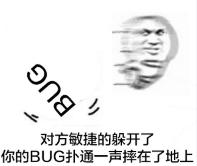# 1. 滴滴面试的内容

## 第一次

• 原型链
• 作用域提升，变量提升
• 执行上下文和执行栈
• 事件循环机制Eventloop
• this的指向问题

• 不用if-else，switch，或>,<等比较大小的符号，总分为100的考试，得到学生的等级(90-100为一等生，80-90为二等生，以此类推)，我说的思路是求余，大佬们在评论区给给思路😃

• leetcode 227题 基本计算器II
• 版本号比较大小(循序渐进的慢慢提出新问题，并解决)

## 简单刨析下面试题

### 1.基本计算器题目

`题目：1 <= s.length <= 3 * 105， s 由整数和算符 ('+', '-', '*', '/') 组成，中间由一些空格隔开 s 表示一个 有效表达式 表达式中的所有整数都是**非负整数**，且在范围 [0, 231 - 1] 内 题目数据保证答案是一个 32-bit 整数。`

``````var calculate = function(s) {
// s=s.trim();//去除两边的空格
const stack=[];//数值栈
let sign='+';//符号
let ans=0;//累加和
s = s.replace(/\s/g, '')
for(let i=0 ; i<s.length ; i++ ){
let num ='';
//判断是否是符号
if(s[i]<'0'){
sign=s[i];
}else{
while(s[i]>='0'&&i<s.length){
num=num+s[i];
i++;//这里是用于组合两位数以上的值
}
i--;//如果上面跳出来了就多了一次，最大是s.length
//接下来，把数据压入栈
num=Number(num);
switch(sign){
case '+':stack.push(num);
break;
//减法化为负数
case '-':stack.push(-num);
break;
//乘除就与栈顶元素进行运算再压入栈
case '*':stack.push(stack.pop()*num);
break;
case '/':
stack.push(stack.pop() / num | 0);
//注意这|的用法，它能得到一个整数
}
}
}
//最后只需要累加
while(stack.length){
ans+=stack.pop();
}
return ans;
};

### 2.项目优化改错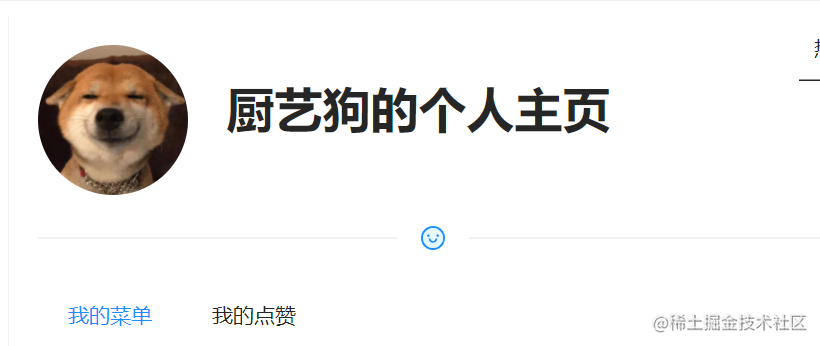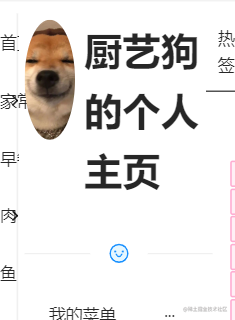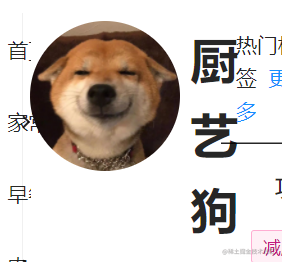`flex-shrink`(定义了项目的缩小比例)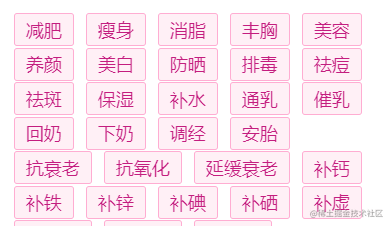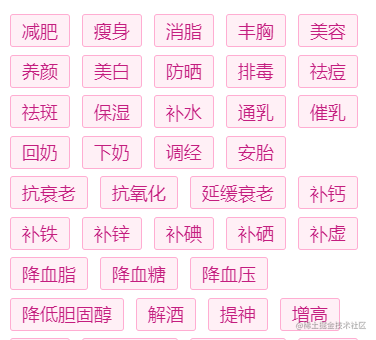### 3.第二次滴滴面试时写的代码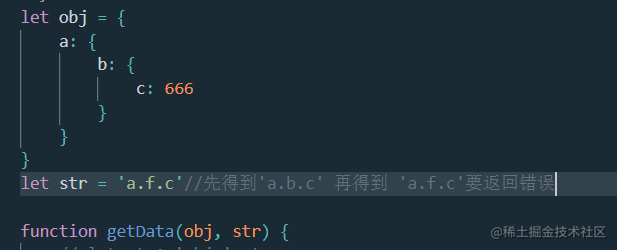• 第一步，面试官叫我通过`'a.b.c'`与obj得到c的值。

``````let obj = {
a: {
b: {
c: 666
}
}
}
let str = 'a.b.c'//先得到'a.b.c' 再得到 'a.f.c'要返回错误

function getData(obj, str) {
let arr=str.split('.');
for(let i=0;i<arr.length;i++){
obj=obj[arr[i]]
}
return obj;
}
console.log(getData(obj, str)) //666

``````function getData(obj, str) {
return eval(`obj.\${str}`);//直接运行字符串，一步到位，太粗暴简单了
}

• 第二步，考虑`a.f.c`这样的情况,即混入不存在的属性。
``````function getData(obj, str) {
let arr=str.split('.');
for(let i=0;i<arr.length;i++){
if(!(obj=obj[arr[i]])){
return false;
};
}
return obj;
}

• 第三步使用es6里的迭代器，比如reduce，将代码写成一行就得到结果
``````function getData(obj, str) {
return str.split('.').reduce((x,y)=>(x?x[y]:x),obj);
//运用三元运算符判断，如果是a.f.c,就返回的是undefined
}

# 2.面试注意点总结

## 介绍项目

`项目怎么来的 + 解决了什么问题，想做成什么 + 说说项目里的需求， + 使用了什么技术栈，采用了什么行动方式，具体做了哪些工作内容 + 有哪些难点，怎么解决的 + 结果如何，有什么体会总结`